Anzeige

# Elect principles -_kirchhoffs_laws

sdacey
16. May 2013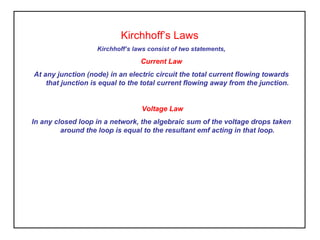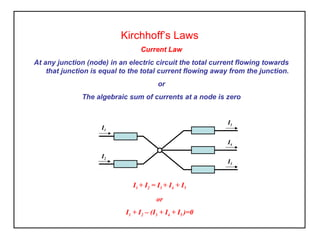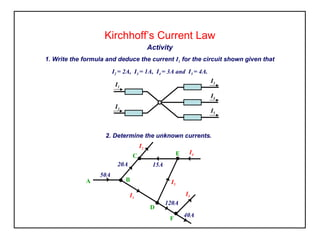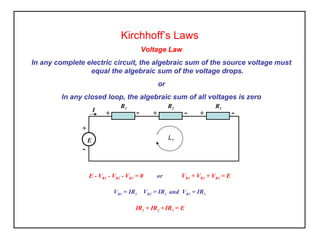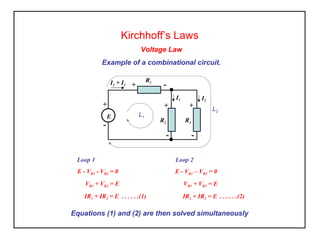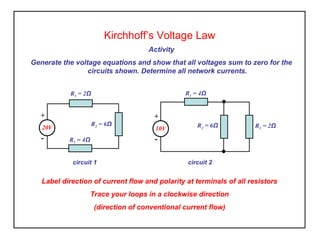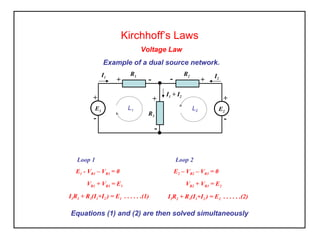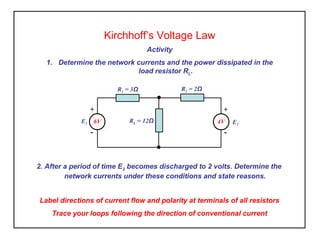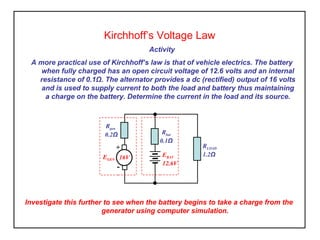1 von 10
Anzeige

### Elect principles -_kirchhoffs_laws

1. Kirchhoff’s Laws Kirchhoff’s laws consist of two statements, Current Law At any junction (node) in an electric circuit the total current flowing towards that junction is equal to the total current flowing away from the junction. Voltage Law In any closed loop in a network, the algebraic sum of the voltage drops taken around the loop is equal to the resultant emf acting in that loop.
2. Kirchhoff’s Laws Current Law At any junction (node) in an electric circuit the total current flowing towards that junction is equal to the total current flowing away from the junction. or The algebraic sum of currents at a node is zero I1 + I2 = I3 + I4 + I5 or I1 + I2 – (I3 + I4 + I5 )=0 I3 I4 I5 I2 I1
3. Kirchhoff’s Current Law Activity 1. Write the formula and deduce the current I1 for the circuit shown given that I2 = 2A, I3 = 1A, I4 = 3A and I5 = 4A. I3 I4 I5 I2 I1 2. Determine the unknown currents. I1 I4 I5 I3 EC B F D A 40A 120A 50A 20A 15A I2
4. Kirchhoff’s Laws Voltage Law In any complete electric circuit, the algebraic sum of the source voltage must equal the algebraic sum of the voltage drops. or In any closed loop, the algebraic sum of all voltages is zero - I + E + + +- - - R2R1 R3 L1 VR1 + VR2 + VR3 = EE - VR1 - VR2 - VR3 = 0 or VR1 = IR1 VR2 = IR2 and VR3 = IR3 IR1 + IR2 + IR3 = E
5. Kirchhoff’s Laws Voltage Law Example of a combinational circuit. L2 + - E + - R2 + - R1 + - R3 I1 + I2 L1 I2 I1 VR1 + VR2 = E E - VR1 - VR2 = 0 IR1 + IR2 = E . . . . . .(1) Loop 1 VR1 + VR3 = E E - VR1 – VR3 = 0 Loop 2 IR1 + IR3 = E . . . . . .(2) Equations (1) and (2) are then solved simultaneously
6. Kirchhoff’s Voltage Law Activity Generate the voltage equations and show that all voltages sum to zero for the circuits shown. Determine all network currents. Label direction of current flow and polarity at terminals of all resistors Trace your loops in a clockwise direction (direction of conventional current flow) - + 20V R1 = 2Ω R3 = 4Ω R2 = 6Ω - + 10V R1 = 4Ω R3 = 2ΩR2 = 6Ω circuit 1 circuit 2
7. Kirchhoff’s Laws Voltage Law Example of a dual source network. Equations (1) and (2) are then solved simultaneously + - E1 + - R3 + - R1 I1 + I2 L1 I2 I1 + - E2 L2 +- R2 VR1 + VR3 = E1 E1 - VR1 – VR3 = 0 I1R1 + R3(I1+I2 ) = E1 . . . . . .(1) Loop 1 VR2 + VR3 = E2 E2 – VR2 – VR3 = 0 Loop 2 I2R2 + R3(I1+I2 ) = E2 . . . . . .(2)
8. Kirchhoff’s Voltage Law Activity 1. Determine the network currents and the power dissipated in the load resistor RL. RL = 12Ω R1 = 3Ω R2 = 2Ω - + 6VE1 E2 - + 4V Label directions of current flow and polarity at terminals of all resistors Trace your loops following the direction of conventional current 2. After a period of time E2 becomes discharged to 2 volts. Determine the network currents under these conditions and state reasons.
9. Kirchhoff’s Voltage Law Activity A more practical use of Kirchhoff’s law is that of vehicle electrics. The battery when fully charged has an open circuit voltage of 12.6 volts and an internal resistance of 0.1Ω. The alternator provides a dc (rectified) output of 16 volts and is used to supply current to both the load and battery thus maintaining a charge on the battery. Determine the current in the load and its source. Investigate this further to see when the battery begins to take a charge from the generator using computer simulation. RLOAD 1.2Ω Rgen 0.2Ω - + EGEN 16V EBAT 12.6V Rbat 0.1Ω
10. Kirchhoff’s Voltage Law Activity A more practical use of Kirchhoff’s law is that of vehicle electrics. The battery when fully charged has an open circuit voltage of 12.6 volts and an internal resistance of 0.1Ω. The alternator provides a dc (rectified) output of 16 volts and is used to supply current to both the load and battery thus maintaining a charge on the battery. Determine the current in the load and its source. Investigate this further to see when the battery begins to take a charge from the generator using computer simulation. RLOAD 1.2Ω Rgen 0.2Ω - + EGEN 16V EBAT 12.6V Rbat 0.1Ω
Anzeige